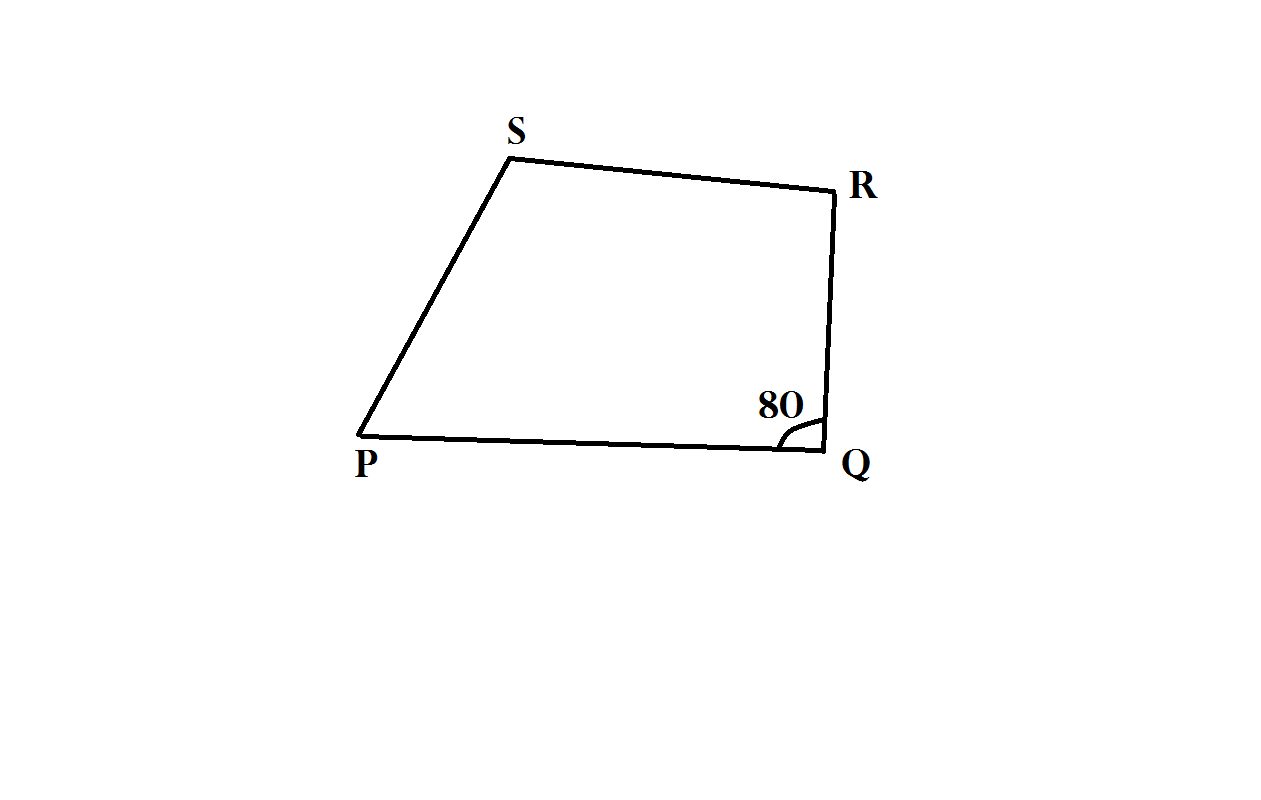# Geometry Quests By Skanda - 4

Geometry Level 1In quadrilateral PQRS, angle Q = 80 degrees.

Angle R - angle S = 50 degrees.

angle P - angle R - angle S = 20 degrees.

Find the measures of angles P, R and S

ASSUME THAT angles P, R and S ARE a, b and c

YOU WILL GET THE ANSWER AS a, b and c

PROVIDE THE ANSWER AS a + b + c

×